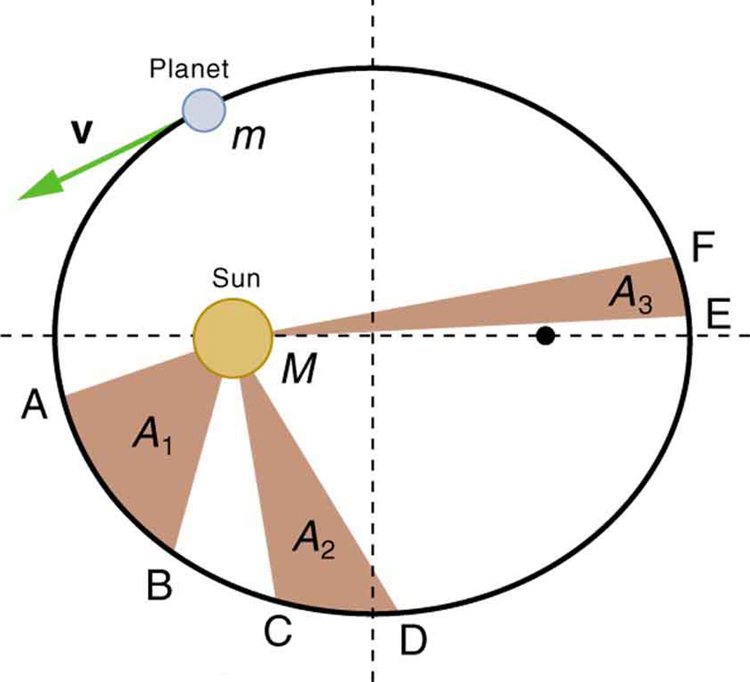# 6.6 Satellites and kepler’s laws: an argument for simplicity  (Page 2/5)

 Page 2 / 5

Kepler’s Third Law

The ratio of the squares of the periods of any two planets about the Sun is equal to the ratio of the cubes of their average distances from the Sun. In equation form, this is

where $T$ is the period (time for one orbit) and $r$ is the average radius. This equation is valid only for comparing two small masses orbiting the same large one. Most importantly, this is a descriptive equation only, giving no information as to the cause of the equality.The shaded regions have equal areas. It takes equal times for m size 12{m} {} to go from A to B, from C to D, and from E to F. The mass m size 12{m} {} moves fastest when it is closest to M size 12{M} {} . Kepler’s second law was originally devised for planets orbiting the Sun, but it has broader validity.

Note again that while, for historical reasons, Kepler’s laws are stated for planets orbiting the Sun, they are actually valid for all bodies satisfying the two previously stated conditions.

## Find the time for one orbit of an earth satellite

Given that the Moon orbits Earth each 27.3 d and that it is an average distance of $3.84×{\text{10}}^{8}\phantom{\rule{0.25em}{0ex}}\text{m}$ from the center of Earth, calculate the period of an artificial satellite orbiting at an average altitude of 1500 km above Earth’s surface.

Strategy

The period, or time for one orbit, is related to the radius of the orbit by Kepler’s third law, given in mathematical form in . Let us use the subscript 1 for the Moon and the subscript 2 for the satellite. We are asked to find ${T}_{2}$ . The given information tells us that the orbital radius of the Moon is ${r}_{1}=3\text{.}\text{84}×{\text{10}}^{8}\phantom{\rule{0.25em}{0ex}}\text{m}$ , and that the period of the Moon is ${T}_{1}=\text{27.3 d}$ . The height of the artificial satellite above Earth’s surface is given, and so we must add the radius of Earth (6380 km) to get ${r}_{2}=\left(\text{1500}+\text{6380}\right)\phantom{\rule{0.25em}{0ex}}\text{km}=\text{7880}\phantom{\rule{0.25em}{0ex}}\text{km}$ . Now all quantities are known, and so ${T}_{2}$ can be found.

Solution

Kepler’s third law is

To solve for ${T}_{2}$ , we cross-multiply and take the square root, yielding

${T}_{2}={T}_{1}{\left(\frac{{r}_{2}}{{r}_{1}}\right)}^{3/2}\text{.}$

Substituting known values yields

$\begin{array}{lll}{T}_{2}& =& \text{27.3 d}×\frac{\text{24.0 h}}{\text{d}}×{\left(\frac{\text{7880 km}}{3.84×{\text{10}}^{5}\phantom{\rule{0.25em}{0ex}}\text{km}}\right)}^{3/2}\\ & =& \text{1.93 h.}\end{array}$

Discussion This is a reasonable period for a satellite in a fairly low orbit. It is interesting that any satellite at this altitude will orbit in the same amount of time. This fact is related to the condition that the satellite’s mass is small compared with that of Earth.

People immediately search for deeper meaning when broadly applicable laws, like Kepler’s, are discovered. It was Newton who took the next giant step when he proposed the law of universal gravitation. While Kepler was able to discover what was happening, Newton discovered that gravitational force was the cause.

## Derivation of kepler’s third law for circular orbits

We shall derive Kepler’s third law, starting with Newton’s laws of motion and his universal law of gravitation. The point is to demonstrate that the force of gravity is the cause for Kepler’s laws (although we will only derive the third one).

Let us consider a circular orbit of a small mass $m$ around a large mass $M$ , satisfying the two conditions stated at the beginning of this section. Gravity supplies the centripetal force to mass $m$ . Starting with Newton’s second law applied to circular motion,

does the force in a system result in the energy transfer?
full meaning of GPS system
how to prove that Newton's law of universal gravitation F = GmM ______ R²
sir dose it apply to the human system
prove that the centrimental force Fc= M1V² _________ r
prove that centripetal force Fc = MV² ______ r
Kaka
how lesers can transmit information
griffts bridge derivative
below me
please explain; when a glass rod is rubbed with silk, it becomes positive and the silk becomes negative- yet both attracts dust. does dust have third types of charge that is attracted to both positive and negative
what is a conductor
Timothy
hello
Timothy
below me
why below you
Timothy
no....I said below me ...... nothing below .....ok?
dust particles contains both positive and negative charge particles
Mbutene
corona charge can verify
Stephen
when pressure increases the temperature remain what?
remains the temperature
betuel
what is frequency
define precision briefly
CT scanners do not detect details smaller than about 0.5 mm. Is this limitation due to the wavelength of x rays? Explain.
hope this helps
what's critical angle
The Critical Angle Derivation So the critical angle is defined as the angle of incidence that provides an angle of refraction of 90-degrees. Make particular note that the critical angle is an angle of incidence value. For the water-air boundary, the critical angle is 48.6-degrees.
okay whatever
Chidalu
pls who can give the definition of relative density?
Temiloluwa
the ratio of the density of a substance to the density of a standard, usually water for a liquid or solid, and air for a gas.
Chidalu
What is momentum
mass ×velocity
Chidalu
it is the product of mass ×velocity of an object
Chidalu
how do I highlight a sentence]p? I select the sentence but get options like copy or web search but no highlight. tks. src
then you can edit your work anyway you wantByByBy Szilárd JankóBy Brooke DelaneyBy Dionne MahaffeyBy Edgar DelgadoBy RhodesBy Madison ChristianBy OpenStaxBy Anonymous UserBy Darlene PaliswatBy Jugnu Khan